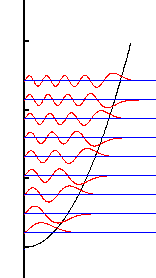# Modified Quantum Harmonic Oscillator

Gabriel Maia
This is more of a conceptual question and I have not had the knowledge to solve it.

We're given a modified quantum harmonic oscillator. Its hamiltonian is

H=$\frac{P^{2}}{2m}$+V(x)

where V(x)=$\frac{1}{2}$m$\omega^{2}x^{2}$ for x$\geq$0 and V(x)=$\infty$ otherwise.

I'm asked to justify in terms of the parity of the quantum problem eigenfunctions why only odd integers n are allowed for the eigenenergies of the problem E=(n+1/2)$\hbar\omega$

I have not a clue... well... actually my best guess was that the given potential impose the condition that the wavefunction is zero at x=0 and this is only the case for the odd ones. I'm confident about it but I could not find a problem like this anywhere to check my answer.

Thank you.

Last edited:

## Answers and Replies